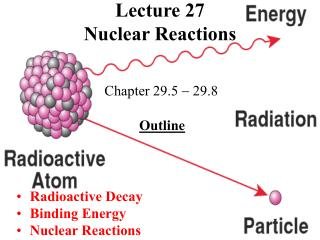DownloadDownload PresentationLecture 27 Nuclear Reactions

# Lecture 27 Nuclear Reactions

Download Presentation## Lecture 27 Nuclear Reactions

- - - - - - - - - - - - - - - - - - - - - - - - - - - E N D - - - - - - - - - - - - - - - - - - - - - - - - - - -
##### Presentation Transcript

1. Lecture 27Nuclear Reactions Chapter 29.5  29.8 Outline • Radioactive Decay • Binding Energy • Nuclear Reactions

2. Half-Life The half-life of a radionuclide is the period of time needed for half of any its initial amount to decay. Radionuclides have unchanging half-life. This is the base for archaeological dating. Half-life for 14C is 5730 years. 146C  147N + e + ¯ ¯  antineutrino Natural abundance ratio of 14C and 12C is ~ 1.31012 Creation of 14C is due to cosmic rays After death, organisms stop absorbing 14C from the atmosphere and the ratio decreases with time.

3. Half-Life

4. Problem with Carbon Isotopes Problem: A 1-gram sample of wood is taken from an ancient site. If the 14C activity of the sample is 12.5% that of the present-day organic material, what is the age of the wood? Solution: Half-time of 14C = 5730 years. 12.5% = 1/8 = 3 half-times of 14C. The sample age is 3*5730 years ~ 17200 years.

5. Binding Energy Hydrogen has 3 isotopes: protium, deuterium, and tritium. The mass of 11H is 1.0078 u , the mass of a neutron is 1.0087 u . The sum is 2.0165 u . The mass of a deuterium atom is 2.0141 u: 0.0024 u = 2.2 MeV less than the combined mass of p+n. All atoms have smaller masses than the sum of masses of their nucleons. Some mass and energy is given off due to action of forces that hold the protons and neutrons together. This energy is called binding energy.

6. Binding Energy Binding energy of a deuterium atom is 2.2 MeV (1.1 MeV per nucleon) , of a 20983Bi is 1640 MeV (7.8 MeV per nucleon). A typical binding energy is ~800 billion kJ/kg To boil water it takes 2260 kJ/kg The most stable element is iron (5626Fe).

7. Problem with Binding Energy What is the binding energy per nucleon of 19779Au? Masses: 19779Au: 196.967 u 11H : 1.0078 10n : 1.0087 1u = 931.5 MeV/c2 Solution: there are 79 p and 118 n in an Au atom. mass(79p+118n) = 198.643 u Difference between the actual mass and  = 1.676 u E = mc2 = 1.676 u * 931.5 (MeV/u) /197 = 7.92MeV

8. Nuclear Reactions Nuclear reactions are changes of elemental composition in atomic interactions. In chemical reactions atomic composition does not change. Elements lighter than Fe can be produced by fusion of 2 smaller nuclei. This is possible, because binding energy increases with atomic number. Fusion requires extremely high temperatures and pressures  fusing nuclei come very close together to overcome Coulomb repulsion forces.

9. Fusion

10. Fission Fission is splitting of a large nucleus into a few lighter nuclei. It is possible for atoms heavier than Fe because the binding energy decreases with the atomic number. Fission does not require extreme conditions and could be easily controlled. The first controlled fission reaction was achieved in 1939 . Fission animation

11. Summary There are two basic types of nuclear reactions: fusion and fission. Fusion is creation heavier elements from lighter ones. It works from hydrogen to iron. Fission is a decay of heavy elements into lighter parts. It works in elements heavier than iron. Fusion is much more energy efficient than fission.